How to make anomalies and plot timeseries (Example: Nino 3.4 Index), and take Composite mean of anomalies for Elnino and Lanina

Authors:

## Lets load the data first.¶

In :
# Run this cell when you are in colab and then restart runtime

!apt-get install libproj-dev proj-data proj-bin
!apt-get install libgeos-dev
!pip install cython
!pip install cartopy

!apt-get -qq install python-cartopy python3-cartopy
!pip uninstall -y shapely    # cartopy and shapely aren't friends (early 2020)
!pip install shapely --no-binary shapely

Reading package lists... Done
Building dependency tree... Done
0 upgraded, 0 newly installed, 0 to remove and 9 not upgraded.
Building dependency tree... Done
0 upgraded, 0 newly installed, 0 to remove and 9 not upgraded.
Requirement already satisfied: cython in /usr/local/lib/python3.10/dist-packages (0.29.36)
Requirement already satisfied: cartopy in /usr/local/lib/python3.10/dist-packages (0.21.1)
Requirement already satisfied: numpy>=1.18 in /usr/local/lib/python3.10/dist-packages (from cartopy) (1.22.4)
Requirement already satisfied: matplotlib>=3.1 in /usr/local/lib/python3.10/dist-packages (from cartopy) (3.7.1)
Requirement already satisfied: shapely>=1.6.4 in /usr/local/lib/python3.10/dist-packages (from cartopy) (2.0.1)
Requirement already satisfied: pyshp>=2.1 in /usr/local/lib/python3.10/dist-packages (from cartopy) (2.3.1)
Requirement already satisfied: pyproj>=3.0.0 in /usr/local/lib/python3.10/dist-packages (from cartopy) (3.6.0)
Requirement already satisfied: contourpy>=1.0.1 in /usr/local/lib/python3.10/dist-packages (from matplotlib>=3.1->cartopy) (1.1.0)
Requirement already satisfied: cycler>=0.10 in /usr/local/lib/python3.10/dist-packages (from matplotlib>=3.1->cartopy) (0.11.0)
Requirement already satisfied: fonttools>=4.22.0 in /usr/local/lib/python3.10/dist-packages (from matplotlib>=3.1->cartopy) (4.41.1)
Requirement already satisfied: kiwisolver>=1.0.1 in /usr/local/lib/python3.10/dist-packages (from matplotlib>=3.1->cartopy) (1.4.4)
Requirement already satisfied: packaging>=20.0 in /usr/local/lib/python3.10/dist-packages (from matplotlib>=3.1->cartopy) (23.1)
Requirement already satisfied: pillow>=6.2.0 in /usr/local/lib/python3.10/dist-packages (from matplotlib>=3.1->cartopy) (9.4.0)
Requirement already satisfied: pyparsing>=2.3.1 in /usr/local/lib/python3.10/dist-packages (from matplotlib>=3.1->cartopy) (3.1.0)
Requirement already satisfied: python-dateutil>=2.7 in /usr/local/lib/python3.10/dist-packages (from matplotlib>=3.1->cartopy) (2.8.2)
Requirement already satisfied: certifi in /usr/local/lib/python3.10/dist-packages (from pyproj>=3.0.0->cartopy) (2023.7.22)
Requirement already satisfied: six>=1.5 in /usr/local/lib/python3.10/dist-packages (from python-dateutil>=2.7->matplotlib>=3.1->cartopy) (1.16.0)
E: Unable to locate package python-cartopy
Found existing installation: shapely 2.0.1
Uninstalling shapely-2.0.1:
Successfully uninstalled shapely-2.0.1
Collecting shapely
Using cached shapely-2.0.1-cp310-cp310-linux_x86_64.whl
Requirement already satisfied: numpy>=1.14 in /usr/local/lib/python3.10/dist-packages (from shapely) (1.22.4)
Installing collected packages: shapely
Successfully installed shapely-2.0.1

In :
import xarray as xr
import matplotlib.pyplot as plt
import numpy as np
import cartopy.crs as ccrs
import matplotlib.pyplot as plt

In :
# from google.colab import drive
# drive.mount('/content/drive')


In :
!wget https://downloads.psl.noaa.gov/Datasets/noaa.oisst.v2/sst.mnmean.nc

--2023-07-29 00:07:59--  https://downloads.psl.noaa.gov/Datasets/noaa.oisst.v2/sst.mnmean.nc
HTTP request sent, awaiting response... 200 OK
Length: 64038940 (61M) [application/x-netcdf]
Saving to: ‘sst.mnmean.nc’

sst.mnmean.nc       100%[===================>]  61.07M  15.2MB/s    in 4.0s

2023-07-29 00:08:04 (15.2 MB/s) - ‘sst.mnmean.nc’ saved [64038940/64038940]


In :
# Read the data using xarray
ds = xr.open_dataset('/content/sst.mnmean.nc')

#ds = xr.decode_cf(ds, use_cftime=True) # Ignore this line. It is only needed if time coordinates are given in floating data and you need to convert it to cftime format
ds

Out:
<xarray.Dataset>
Dimensions:    (lat: 180, lon: 360, time: 494, nbnds: 2)
Coordinates:
* lat        (lat) float32 89.5 88.5 87.5 86.5 ... -86.5 -87.5 -88.5 -89.5
* lon        (lon) float32 0.5 1.5 2.5 3.5 4.5 ... 356.5 357.5 358.5 359.5
* time       (time) datetime64[ns] 1981-12-01 1982-01-01 ... 2023-01-01
Dimensions without coordinates: nbnds
Data variables:
sst        (time, lat, lon) float32 ...
time_bnds  (time, nbnds) datetime64[ns] ...
Attributes:
title:          NOAA Optimum Interpolation (OI) SST V2
Conventions:    CF-1.0
history:        Wed Apr  6 13:47:45 2005: ncks -d time,0,278 SAVEs/sst.mn...
comments:       Data described in  Reynolds, R.W., N.A. Rayner, T.M.\nSmi...
platform:       Model
source:         NCEP Climate Modeling Branch
institution:    National Centers for Environmental Prediction
References:     https://www.psl.noaa.gov/data/gridded/data.noaa.oisst.v2....
dataset_title:  NOAA Optimum Interpolation (OI) SST V2
source_url:     http://www.emc.ncep.noaa.gov/research/cmb/sst_analysis/
In :
# Choose the SST variable store it's average
tos = ds.sst
average_sst = tos.mean(dim='time')
del ds

In :
fig, (ax) = plt.subplots(
ncols=1, nrows=1, figsize=[8, 4], subplot_kw={"projection": ccrs.Robinson()}
)

# plot the data
average_sst.plot(
ax=ax,
x="lon",
y="lat",
transform=ccrs.PlateCarree(),
vmin=-1,
vmax=30,
cmap="coolwarm",
robust=True,
)
ax.coastlines()
ax.set_global()
ax.set_title("Average Sea Surface Temperature (°C)")

Out:
Text(0.5, 1.0, 'Average Sea Surface Temperature (°C)')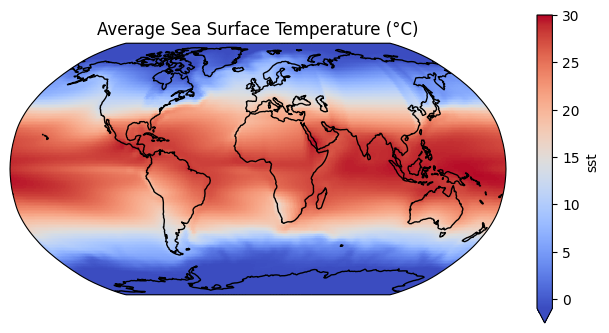## Computing anomalies¶

In this tutorial we will learn about how to deal with time series data. In order to do that, we have chosen a phenomenon called El Nino Southern Oscillation (ENSO). It is a climate pattern in the pacific ocean and can affect weather pattern worldwide. Have a go through this link: https://oceanservice.noaa.gov/facts/ninonina.html

In :
# data has been grouped by month
gb = tos.groupby('time.month')
# calculating anomaly
tos_anom = gb - gb.mean(dim='time')

In :
#ploting 2016 january anomalies which is an elnino year.
tos_anom_at_time = tos_anom.sel(time='2016-01-01')
print(tos_anom_at_time.min())
print(tos_anom_at_time.max())

<xarray.DataArray 'sst' ()>
array(-3.3045235, dtype=float32)
Coordinates:
time     datetime64[ns] 2016-01-01
month    int64 1
<xarray.DataArray 'sst' ()>
array(3.569044, dtype=float32)
Coordinates:
time     datetime64[ns] 2016-01-01
month    int64 1

In :
# Select the temperature anomaly at the chosen time
tos_anom_at_time = tos_anom.sel(time='2016-01-01')

lon = tos_anom_at_time.lon
lat = tos_anom_at_time.lat

fig, (ax) = plt.subplots(
ncols=1, nrows=1, figsize=[8, 4], subplot_kw={"projection": ccrs.Robinson()}
)

# plot the data
tos_anom_at_time.plot(
ax=ax,
x="lon",
y="lat",
transform=ccrs.PlateCarree(),
vmin=-4,
vmax=4,
cmap="coolwarm",
robust=True,
)
ax.coastlines()
ax.set_global()
ax.set_title('Sea Surface Temperature Anomaly at Time: {}'.format(tos_anom_at_time.time.values), pad=20)

Out:
Text(0.5, 1.0, 'Sea Surface Temperature Anomaly at Time: 2016-01-01T00:00:00.000000000')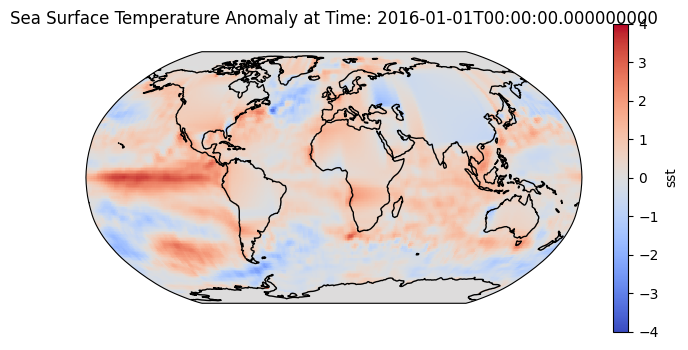In :
# Now we will calculate the mean global anomaly by averaging over all the lats and lons

unweighted_mean_global_anom = tos_anom.mean(dim=['lat','lon'])
unweighted_mean_global_anom.plot()

Out:
[<matplotlib.lines.Line2D at 0x7bf9eb207c10>]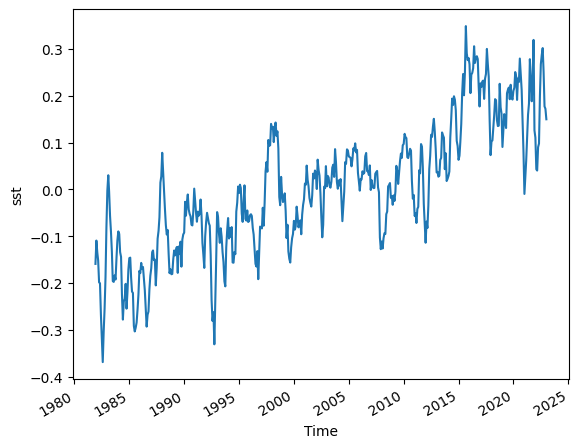## As we move toward the poles, each grid cell covers less area. So we need to account for that when calculating mean global anomaly. This is called weighted average and we will use the grid cell area as weights. If the dataset doesn't include any variable containing area of the cells, you can apply the method below which is using the latitudes to calculate the area of the grid cells.¶

In :
# For a rectangular grid the cosine of the latitude is proportional to the grid cell area.
weights.name = "weights"

In :
# Calculating the weighted global anomaly using the 'weights' we have created
weighted_mean_global_anom = tos_anom.weighted(weights).mean(dim=['lat','lon'])

In :
# Plotting weighted and unweighted mean together to see difference
unweighted_mean_global_anom.plot(size=5)
weighted_mean_global_anom.plot()
plt.legend(['unweighted','weighted'])

Out:
<matplotlib.legend.Legend at 0x7bf9eb07c370>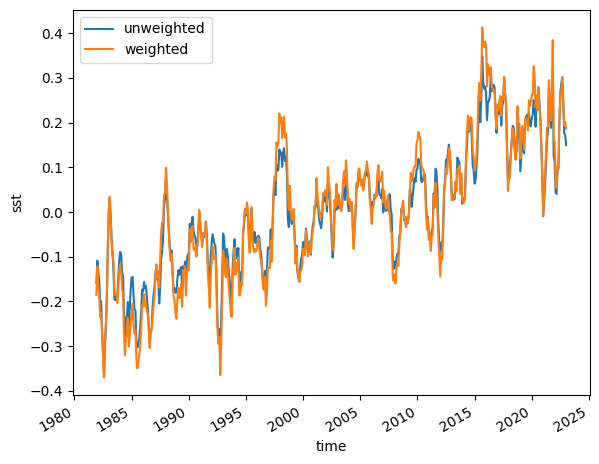## Now we will calculate rolling mean. A rolling mean helps to reduce the impact of short-term fluctuations or noise in the data. It provides a clearer representation of the underlying trend or pattern by averaging out these variations over a specified window.¶

In :
# Using rolling method to compute averages in a moving window of 5-months of data:

m_avg = weighted_mean_global_anom.rolling(time=5, center=True).mean()

In :
# Now using the methods we will calculate and plot Nino 3.4 Index
# First we will subset our data (for Nino 3.4 region) using two methods
# Slice

tos_nino34 = tos.sel(lat=slice(-5,5), lon=slice(190,240))

tos_nino34 = tos.where((tos.lat>-5) & (tos.lat<5) & (tos.lon>190) & (tos.lon<240), drop=True)

In :
# Here we are repeating the computation we learned above for calculating anomalies. This time for the Nino 3.4 area.

gb2 = tos_nino34.groupby('time.month')
nino34_anom = gb2 - gb2.mean(dim='time')
index_nino34 = nino34_anom.mean(dim=['lat','lon'])
index_nino34_rolling_mean = index_nino34.rolling(time=5, center=True).mean()

In :
# Let's plot mean anomaly and 5-month running mean anomaly to compare

index_nino34.plot(size=6)
index_nino34_rolling_mean.plot()
plt.legend(['anomaly', '5-month running mean anomaly'])
plt.title('SST anomaly over the Niño 3.4 region');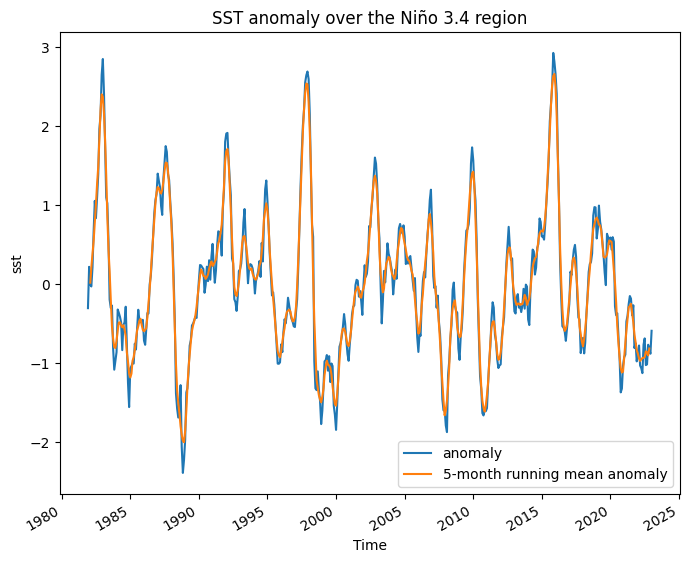In :


In :


In :
## routines for calculating seasonal climatology and seasonal timeseries
import xarray as xr
import numpy as np

dpm = {'noleap': [0, 31, 28, 31, 30, 31, 30, 31, 31, 30, 31, 30, 31],
'365_day': [0, 31, 28, 31, 30, 31, 30, 31, 31, 30, 31, 30, 31],
'standard': [0, 31, 28, 31, 30, 31, 30, 31, 31, 30, 31, 30, 31],
'gregorian': [0, 31, 28, 31, 30, 31, 30, 31, 31, 30, 31, 30, 31],
'proleptic_gregorian': [0, 31, 28, 31, 30, 31, 30, 31, 31, 30, 31, 30, 31],
'all_leap': [0, 31, 29, 31, 30, 31, 30, 31, 31, 30, 31, 30, 31],
'366_day': [0, 31, 29, 31, 30, 31, 30, 31, 31, 30, 31, 30, 31],
'360_day': [0, 30, 30, 30, 30, 30, 30, 30, 30, 30, 30, 30, 30]}

def leap_year(year, calendar='standard'):
"""Determine if year is a leap year
Args:
year (numeric)
"""
leap = False
if ((calendar in ['standard', 'gregorian',
'proleptic_gregorian', 'julian']) and
(year % 4 == 0)):
leap = True
if ((calendar == 'proleptic_gregorian') and
(year % 100 == 0) and
(year % 400 != 0)):
leap = False
elif ((calendar in ['standard', 'gregorian']) and
(year % 100 == 0) and (year % 400 != 0) and
(year < 1583)):
leap = False
return leap

def get_days_per_mon(time, calendar='standard'):
"""
return a array of days per month corresponding to the months provided in months

Args: time (CFTimeIndex): ie. ds.time.to_index()
calendar (str): default 'standard'
"""
month_length = np.zeros(len(time), dtype=np.int)

cal_days = dpm[calendar]

for i, (month, year) in enumerate(zip(time.month, time.year)):
month_length[i] = cal_days[month]
if leap_year(year, calendar=calendar):
month_length[i] += 1
return month_length

def season_mean(ds, season = "all", cal = "none"):
""" calculate climatological mean by season
Args: ds (xarray.Dataset): dataset
var (str): variable to use
season (str): "all", 'DJF', "MAM", "JJA", "SON"
cal (str): "none"(default) or calendar used for weighting months by number of days
"""
## no weighting of months:
if cal == "none":
if season == "all":
## calculate mean for all season
smean = ds.groupby('time.season').mean('time')
else :
## calculate mean for specified season
smean = ds.where(ds['time.season'] == season).mean('time')

return smean
## weighted months
else:
## create array of month_length (number of days in each month)
## assign time coords matching original ds
month_length = xr.DataArray(get_days_per_mon(ds.time.to_index(), calendar=cal),
coords=[ds.time], name='month_length')
## Calculate the weights by grouping by 'time.season'
weights = month_length.groupby('time.season') / month_length.groupby('time.season').sum()

if season == "all":
## calculate weighted mean for all season
smean = (ds * weights).groupby('time.season').mean('time')
else :
## calculate weighted mean for specified season
smean = (ds * weights).where(ds['time.season'] == season).mean('time')

return smean[season]

def season_ts(ds, season):
""" calculate timeseries of seasonal averages
Args: ds (xarray.Dataset): dataset
var (str): variable to calculate
season (str): 'DJF', 'MAM', 'JJA', 'SON'
"""
## set months outside of season to nan
ds_season = ds.where(ds['time.season'] == season)

# calculate 3month rolling mean (only middle months of season will have non-nan values)
ds_season = ds_season.rolling(min_periods=3, center=True, time=3).mean()

# reduce to one value per year
ds_season = ds_season.groupby('time.year').mean('time')

# FUTURE: remove first year if it has nan?
return ds_season

In :
del gb

In :
# creating nino34 index sst timeseries for DJF month only
tos_djf_anom_global=season_ts(tos_anom,'DJF')
tos_nino34_djf_anom=tos_djf_anom_global.sel(lat=slice(5,-5),lon=slice(190,240)).mean(dim=['lat','lon'])

In :


In :


In :
# Now let's plot the Nino 3.4 Index

fig = plt.figure(figsize=(12, 6))

tos_nino34_djf_anom.plot(color='black')
plt.axhline(0, color='black', lw=0.5)
plt.axhline(0.5, color='black', linewidth=0.5, linestyle='dotted')
plt.axhline(-0.5, color='black', linewidth=0.5, linestyle='dotted')
plt.title('Niño 3.4 Index');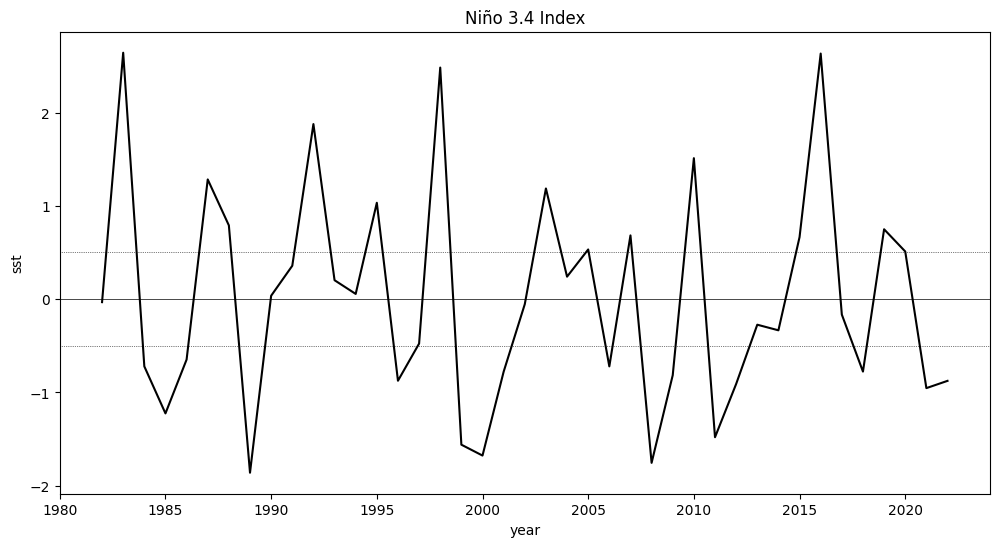In :
## find elnino years

elnino_year=tos_nino34_djf_anom.year[tos_nino34_djf_anom.data>0.5]

## find lanina years

lanina_year=tos_nino34_djf_anom.year[tos_nino34_djf_anom.data<-0.5]

print('elnino, ',elnino_year.data)
print('lanina, ',lanina_year.data)

elnino,  [1983 1987 1988 1992 1995 1998 2003 2005 2007 2010 2015 2016 2019 2020]
lanina,  [1984 1985 1986 1989 1996 1999 2000 2001 2006 2008 2009 2011 2012 2018
2021 2022]

In :
# elnino anomaly compoite mean DJF

elnino_anom_compmean=tos_djf_anom_global.sel(year=elnino_year.data).mean(dim='year')

# lanina anomaly compoite mean DJF

lanina_anom_compmean=tos_djf_anom_global.sel(year=lanina_year.data).mean(dim='year')

In :
fig, (ax) = plt.subplots(
ncols=1, nrows=3, figsize=[10, 12], subplot_kw={"projection": ccrs.Robinson()}
)

# Elnino
elnino_anom_compmean.plot(
ax=ax,
x="lon",
y=&q